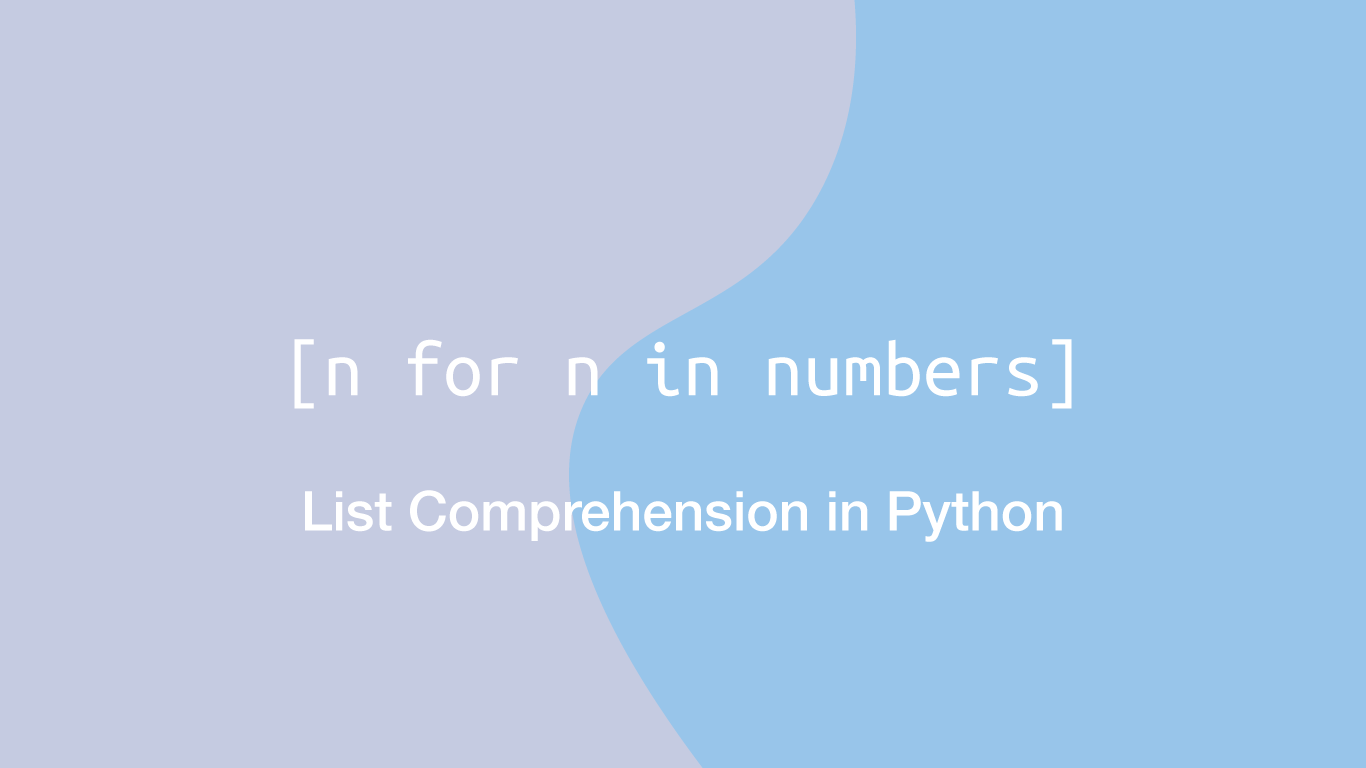# How to Use Python List ComprehensionEssentially, list comprehension in Python is a cleaner way of creating lists from iterable data types. In this tutorial, we will learn how to create lists using list comprehension and when using a more traditional approach might make more sense.

## Creating a List with a For Loop

Before we jump into list comprehension let's look at how we might build a `list` from an iterable such as a string. In the example below, we are iterating through the string `hello` and adding each value to a list using the `append()` method.

``````items = []
string = 'hello'

for c in string:
items.append(c)

print(items)
``````
``````['h', 'e', 'l', 'l', 'o']
``````

In the above code, we are creating an empty `list`, then using a `for` loop we are iterating through each character in the string and appending it to the list before printing the populated `list`.

## Creating a List Using List Comprehension

The above example is perfectly good Python code, though we can do the same thing on one line using list comprehension.

``````string = 'hello'

items = [c for c in string]

print(items)
``````
``````['h', 'e', 'l', 'l', 'o']
``````

In the example above we are creating a new `list` and adding values to it by running a `for` loop on an iterable within it. That is essentially how list comprehension works.

## List Comprehension with Tuples, Dictionaries

So far we have only used strings to demonstrate list comprehensions, but it is possible to use other iterable data types with list comprehensions such as tuples, dictionaries .etc. Let's try a couple more examples using different types of iterables and see what the output is.

Tuple to list:

``````tup = (1,3,4,6)

items = [c for c in tup]

print(items)
``````
``````[1, 3, 4, 6]
``````

Dictionary to list:

``````d = {'apples': 1, 'oranges': 2}

items = [[c,str(i)] for c, i in d.items()]

print(items)
``````
``````[['apples', '1'], ['oranges', '2']]
``````

## List Comprehension with Conditional Statements

It is possible to use conditional statements with list comprehensions by placing the logic after the `for` loop (which will run like it was inside of the `for` loop on each iteration.) If the statement returns `True` the value will be added to the `list`.

``````string = 'hello'

items = [c for c in string if c != 'h']

print(items)
``````
``````['e', 'l', 'l', 'o']
``````

## Nested IF Statements

To nest `if` statements place one `if` statement after the other. In the example below, we are checking if the value is less than `6` then checking if it is greater than `1` – if both conditionals return `True` the value will be added to the new `list`.

``````tup = (1,3,4,6)

items = [c for c in tup if c < 6 if c > 1]

print(items)
``````
``````[3, 4]
``````

## IF ELSE Statements

An `else` statement must be put in front of the `for` loop because we are changing what value is added to the new `list` depending on the result of a conditional.

``````tup = (1,3,4,6)

items = [c if c < 6 else 1 for c in tup]

print(items)
``````
``````[1, 3, 4, 1]
``````

In the example above we are saying if the value is less than `6` add it, else add `1` instead.

## Nested List Comprehension Loops

It is possible to nest list comprehensions inside parent list comprehensions like this:

``````items = [[d for d in range(0,3)] for n in range(0,9)]

print(items)
``````
``````[[0, 1, 2], [0, 1, 2], [0, 1, 2], [0, 1, 2], [0, 1, 2], [0, 1, 2], [0, 1, 2], [0, 1, 2], [0, 1, 2]]
``````

In the above example, we are building a list of lists using a range of numbers.

## When Should I Use List Comprehension?

It is subjective. The whole point of list comprehensions is to make the code smaller while still being readable – you will have to judge whether things are looking too complex. For building lists that require little or no logic, list comprehension is the way to go. When building nested lists using lots of logic, the regular `for` loop approach is probably going to be more readable.

## Conclusion

You now know how to use list comprehension in Python to build lists in a variety of different ways and when you might choose not to use them.

#### Related Tutorials### How to Round Numbers Up and Down in Python

September 28, 2020### How to Calculate Mean, Median, Mode and Range in Python

October 07, 2020### Convert a List to Lowercase in Python

September 21, 2021### How to Create Range of Floats in Python

September 15, 2021### How to Convert a List to a String in Python

September 17, 2020April 13, 2021